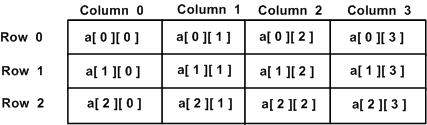# C++ Multi-dimensional Arrays

C++ allows multidimensional arrays. Here is the general form of a multidimensional array declaration −

```type name[size1][size2]...[sizeN];
```

For example, the following declaration creates a three dimensional 5 . 10 . 4 integer array −

```int threedim;
```

## Two-Dimensional Arrays

The simplest form of the multidimensional array is the two-dimensional array. A two-dimensional array is, in essence, a list of one-dimensional arrays. To declare a two-dimensional integer array of size x,y, you would write something as follows −

```type arrayName [ x ][ y ];
```

Where type can be any valid C++ data type and arrayName will be a valid C++ identifier.

A two-dimensional array can be think as a table, which will have x number of rows and y number of columns. A 2-dimensional array a, which contains three rows and four columns can be shown as below −Thus, every element in array a is identified by an element name of the form a[ i ][ j ], where a is the name of the array, and i and j are the subscripts that uniquely identify each element in a.

## Initializing Two-Dimensional Arrays

Multidimensioned arrays may be initialized by specifying bracketed values for each row. Following is an array with 3 rows and each row have 4 columns.

```int a = {
{0, 1, 2, 3} ,   /*  initializers for row indexed by 0 */
{4, 5, 6, 7} ,   /*  initializers for row indexed by 1 */
{8, 9, 10, 11}   /*  initializers for row indexed by 2 */
};
```

The nested braces, which indicate the intended row, are optional. The following initialization is equivalent to previous example −

```int a = {0,1,2,3,4,5,6,7,8,9,10,11};
```

## Accessing Two-Dimensional Array Elements

An element in 2-dimensional array is accessed by using the subscripts, i.e., row index and column index of the array. For example −

```int val = a;
```

The above statement will take 4th element from the 3rd row of the array. You can verify it in the above digram.

```#include <iostream>
using namespace std;

int main () {
// an array with 5 rows and 2 columns.
int a = { {0,0}, {1,2}, {2,4}, {3,6},{4,8}};

// output each array element's value
for ( int i = 0; i < 5; i++ )
for ( int j = 0; j < 2; j++ ) {

cout << "a[" << i << "][" << j << "]: ";
cout << a[i][j]<< endl;
}

return 0;
}
```

When the above code is compiled and executed, it produces the following result −

```a: 0
a: 0
a: 1
a: 2
a: 2
a: 4
a: 3
a: 6
a: 4
a: 8
```

As explained above, you can have arrays with any number of dimensions, although it is likely that most of the arrays you create will be of one or two dimensions.

cpp_arrays.htm